# Linear imaginary equation

Given that
$2\left(z+i\right)=i\left(z+i\right)$
"this is z star" Find the value of the complex number z.

Result

z = (Correct answer is: -i)### Step-by-step explanation: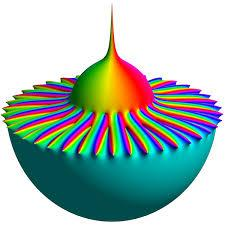Did you find an error or inaccuracy? Feel free to write us. Thank you!Tips to related online calculators
Do you have a linear equation or system of equations and looking for its solution? Or do you have a quadratic equation?
Try our complex numbers calculator.

## Related math problems and questions:

• Distance two imaginary numbs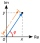Find the distance between two complex number: z1=(-8+i) and z2=(-1+i).
• Complex number coordinatesWhich coordinates show the location of -2+3i
• Modulus and argumentFind the mod z and argument z if z=i
• Imaginary numbersFind two imaginary numbers whose sum is a real number. How are the two imaginary numbers related? What is its sum?
• Log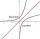Calculate value of expression log |3 +7i +5i2| .
• The modulus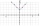Find the modulus of the complex number 2 + 5i
• Evaluate 18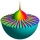Evaluate the expression (-4-7i)-(-6-9i) and write the result in the form a+bi (Real + i* Imaginary).
• ReciprocalCalculate reciprocal of z=0.8-1.8i:
• Mappings of complex numbersFind the images of the following points under mappings: z=3-2j w=2zj+j-1
• De Moivre's formula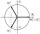There are two distinct complex numbers z, such that z3 is equal to 1 and z is not equal to 1. Calculate the sum of these two numbers.
• Find x 2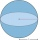Find x, y, and z such that x³+y³+z³=k, for each k from 1 to 100. Write down the number of solutions.
• Z9–I–1In all nine fields of given shape to be filled natural numbers so that: • each of the numbers 2, 4, 6, and 8 is used at least once, • four of the inner square boxes containing the products of the numbers of adjacent cells of the outer square, • in the cir
• Subtracting complex in polar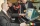Given w =√2(cosine (p/4) + i sine (pi/4) ) and z = 2 (cosine (pi/2) + i sine (pi/2) ), what is w - z expressed in polar form?
• ABS CNCalculate the absolute value of complex number -15-29i.
• Linear combination of complexIf z1=5+3i and z2=4-2i, write the following in the form a+bi a) 4z1+6z2 b) z1*z2
• Two-digit numberDigit sum of thinking two-digit natural number is 11. When it exchanging a sequence of digits, given a number which is 27 less than the thinking number. Find out which number I think.
• Two numbersWe have two numbers. Their sum is 140. One-fifth of the first number is equal to half the second number. Determine those unknown numbers.